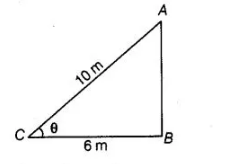# Foot of a 10 m long ladder leaning against

Question:

Foot of a 10 m long ladder leaning against a vertical wall is 6 m away from the base of the wall. Find the height of the point on the wall where the top of

Solution:

Let AB be a vertical wall and AC = 10 m is a ladder. The top of the ladder reaches to A and distance of ladder from the base of the wall BC is 6 m.In right angled $\triangle A B C, \quad A C^{2}=A B^{2}+B C^{2} \quad$ [by Pythagoras theorem]

$\Rightarrow \quad \cdot \quad(10)^{2}=A B^{2}+(6)^{2}$

$\Rightarrow \quad 100=A B^{2}+36$

$\Rightarrow \quad A B^{2}=100-36=64$

$\therefore \quad A B=\sqrt{64}=8 \mathrm{~cm}$

Hence, the height of the point on the wall where the top of the ladder reaches is 8 cm.